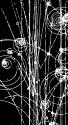# Questions about E = mc^2

• 6 Replies
• 626 Views#### Trekky0623

• Official Member
• 10061##### Questions about E = mc^2
« on: May 30, 2007, 07:42:45 AM »
Some questions about E = mc2
1. If mass can be converted into energy, can energy be converted into mass?  Does this mean that mass and energy are the same things?
2. Why is c relevant to the conversion of mass to energy anyway?  Why do you multiply mass by c2?
3. What must you do to get energy from mass?
Just curious.

?

#### Agent_0042

• 1419##### Re: Questions about E = mc^2
« Reply #1 on: May 30, 2007, 08:23:50 AM »
1. Yes, it can go both ways. However, it is less common for energy to go to mass, for the reason in #2.
2. The C represents the speed of light. You multiply by c2 because a small amount of mass will convert into a tremendous amount of energy. The opposite is also true.
3. Umm, anything? The equation is universal, applying to any instance where mass is converted into energy.

I don't have time to go into detail, so I hope my 30-second-response answers your questions.
Quote
Can the FAQ...
Yes, it can.#### Trekky0623

• Official Member
• 10061##### Re: Questions about E = mc^2
« Reply #2 on: May 30, 2007, 02:11:19 PM »
So does c2 only represent a huge unknown number, or do you actually have to use the value for c?#### sokarul

• 17785
• Discount Chemist##### Re: Questions about E = mc^2
« Reply #3 on: May 30, 2007, 02:14:38 PM »
Nuclear bombs use E=mc2.
C squared is the speed of light squared.
ANNIHILATOR OF  SHIFTER

It's no slur if it's fact.#### TheEngineer

• Planar Moderator
• 15483
• GPS does not require satellites.##### Re: Questions about E = mc^2
« Reply #4 on: May 30, 2007, 02:41:06 PM »
E=mc^2 is derived from the relativistic energy equations.  Since it is relativistic, it contains gamma, or 1/(sqrt(1-u^2/c^2)).  However, the rest mass part of the equation is E=mc^2.  With c being the speed of light."I haven't been wrong since 1961, when I thought I made a mistake."
-- Bob Hudson#### Roundy the Truthinessist

• Flat Earth TheFLAMETHROWER!
• The Elder Ones
• 27043
• I'm the boss.##### Re: Questions about E = mc^2
« Reply #5 on: May 30, 2007, 02:52:12 PM »
This is very interesting.  One thing I've wondered is how it was derived that the speed of light squared would be part of the equation.
Where did you educate the biology, in toulet?#### TheEngineer

• Planar Moderator
• 15483
• GPS does not require satellites.##### Re: Questions about E = mc^2
« Reply #6 on: May 30, 2007, 02:59:17 PM »
To understand the outcome of the Michelson-Morley experiment, Lorenz theorized that the length must contract by a factor of sqrt(1-u^2/c^2) in the direction of motion.  Thus agreeing with the invariant Maxwell equations, which seemed to state that there was no single reference system in which the speed of light is c."I haven't been wrong since 1961, when I thought I made a mistake."
-- Bob Hudson